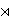### Boris Širola

Department of Mathematics, University of Zagreb, Bijenička 30, 10000 Zagreb, Croatia
e-mail: sirola@math.hr

Abstract.   Let K be a field, char(K) ≠ 2. Suppose G=G(K) is the group of K-points of a reductive algebraic K-group G. Let G1≤ G be the group of K-points of a reductive subgroup G1G. We study the structure of the normalizer N= NG(G1). In particular, let G= SL(2n, K) for n>1. For certain well known embeddings of G1 into G, where G1= Sp(2n, K) or SO(2n, K), we show that N/G1μk(K), the group of k-th roots of unity in K. Here, k=2n if certain Condition (◊) holds, and k=n if not. Moreover, there is a precisely defined subgroup N' of N such that N/N' ≅ Z/2 Z if Condition (◊) holds, and N=N' if not. Furthermore, when n>1, as the main observations of the paper we have the following: (i) N is a self-normalizing subgroup of G; (ii) N' ≅ G1Z[X] μn (K), the semidirect product of G1 by μn (K). Besides we point out that analogous results will hold for a number of other pairs of groups (G,G1). We also show that for the pair (g, g1), of the corresponding K-Lie algebras, g1 is self-normalizing in g; which generalizes a well-known result in the zero characteristic.

2000 Mathematics Subject Classification.   20E34, 20G15, 17B05, 17B20.

Key words and phrases.   Normalizer, self-normalizing subgroup, symmetric pair, symplectic group, even orthogonal group.

Full text (PDF) (access from subscribing institutions only)

DOI: 10.3336/gm.46.2.10

References:

1. E. Artin, Geometric Algebra, Interscience, New York, 1957.
MathSciNet

2. A. Borel, Linear Algebraic groups. Second Enlarged Edition, Graduate Texts in Math., Vol. 126, Springer-Verlag, New York, 1991.
MathSciNet

3. N. Bourbaki, Lie groups and Lie algebras, Chapters 1-3, Springer-Verlag, Berlin, 1989.
MathSciNet

4. N. Bourbaki, Groupes et Algèbres de Lie, Chapitres VII, VIII, Hermann, Paris, 1975.

5. R. Brylinski and B. Kostant, Nilpotent orbits, normality, and Hamiltonian group actions, J. Amer. Math. Soc. 7 (1994), 269-298.
MathSciNet     CrossRef

6. R. Carter, Finite groups of Lie type. Conjugacy classes and complex characters, Wiley-Interscience, Chichester, 1993.
MathSciNet

7. C. Chevalley, Sur certains groupes simples, Tôhoku Math. J (2) 7 (1955), 14-66.
MathSciNet     CrossRef

8. J. Dixmier, Enveloping Algebras, Graduate Studies in Mathematics 11, Amer. Math. Soc., 1996.
MathSciNet

9. E. B. Dynkin, Semisimple subalgbras of semisimple Lie algebras, Amer. Math. Soc. Translations (2) 6 (1957), 111-244.

10. E. B. Dynkin, Maximal subgroups of the classical groups, Amer. Math. Soc. Translations (2) 6 (1957), 245-378.

11. R. Goodman and N. R. Wallach, Representations and Invariants of the Classical Groups, Encyclopedia of Mathematics and its Applications, Vol. 68, Cambridge Univ. Press, Cambridge, 1998.
MathSciNet

12. R. Howe, E.-C. Tan and J. F. Willenbring, Stable branching rules for classical symmetric pairs, Trans. Amer. Math. Soc. 357 (2005), 1601-1626.
MathSciNet     CrossRef

13. J. E. Humphreys, Conjugacy Classes in Semisimple Algebraic Groups, Mathematical Surveys and Monographs, Vol. 43, Amer. Math. Soc., Providence, 1995.
MathSciNet

14. J. C. Jantzen, Nilpotent orbits in representation theory, Lie Theory, Progr. Math., Vol. 228, Birkhäuser, Boston (2004), pp. 1-211.
MathSciNet

15. A. W. Knapp, Geometric interpretations of two branching theorems of D. E. Littlewood, J. Algebra 270 (2003), 728-754.
MathSciNet     CrossRef

16. T. Kobayashi, Discrete decomposability of the restriction of A q(λ ) with respect to reductive subgroups III. Restriction of Harish-Chandra modules and associated varieties, Invent. Math. 131 (1998), 229-256.
MathSciNet     CrossRef

17. T. Kobayashi, Discretely decomposable restrictions of unitary representations of reductive Lie groups-examples and conjectures, Analysis on homogeneous spaces and representation theory of Lie groups, Okayama-Kyoto (1997), 99-127, Adv. Stud. Pure Math. 26, Math. Soc. Japan, Tokyo, 2000.
MathSciNet

18. N. Koblitz, A course in number theory and cryptography, 2nd ed., Graduate Texts in Math., Vol. 114, Springer-Verlag, Berlin, 1994.
MathSciNet

19. B. Kostant, A branching law for subgroups fixed by an involution and a noncompact analogue of the Borel-Weil theorem, Noncommutative harmonic analysis, Progress in Math., vol. 220, Birkhäuser Boston, Boston, 2004, pp 291-353.
MathSciNet

20. T. Levasseur and S. P. Smith, Primitive ideals and nilpotent orbits in type G2, J. Algebra 114 (1988), 81-105.
MathSciNet     CrossRef

21. R. W. Richardson, Jr., Conjugacy classes in Lie algebras and algebraic groups, Ann. of Math. (2) 86 (1967), 1-15.
MathSciNet     CrossRef

22. G. M. Seitz, The maximal subgroups of classical algrebraic groups, Mem. Amer. Math. Soc., 67 No. 365 (1987).
MathSciNet

23. B. Širola, On pairs of complex Lie groups and generalized global Cartan decomposition, Grazer Math. Ber. 348 (2005), 79-90.
MathSciNet

24. B. Širola, A generalized global Cartan decomposition: a basic example, Comm. Algebra 34 (2006), 3267-3279.
MathSciNet     CrossRef

25. B. Širola, Pairs of semisimple Lie algebras and their maximal reductive subalgebras, Algebr. Represent. Theory 11 (2008), 233-250.
MathSciNet     CrossRef

26. B. Širola, Pairs of Lie algebras and their self-normalizing reductive subalgebras, J. Lie Theory 19 (2009), 735-766.
MathSciNet

27. B. Širola, Normalizers and self-normalizing subgroups II, Cent. Eur. J. Math. 9 (2011), 1317-1332.
CrossRef

28. B. Širola, On centralizers and normalizers for groups, preprint.

29. T. A. Springer and R. Steinberg, Conjugacy classes, Seminar on Algebraic Groups and Related Finite Groups (A. Borel et al. eds.), Lecture Notes in Math., Vol. 131, Springer-Verlag, Berlin, 1970, pp. 167-266.
MathSciNet

30. D. A. Vogan, Jr., The unitary dual of G2, Invent. Math. 116 (1994), 677-791.
MathSciNet     CrossRef

31. A. Wagner, On the clssification of the classical groups, Math. Zeit. 97 (1967), 66-76.
MathSciNet     CrossRef

32. G. E. Wall, On the conjugacy classes in the unitary, symplectic and orthogonal groups, J. Austral. Math. Soc. 3 (1963), 1-62.
MathSciNet     CrossRef